# Calculate Time Differences in Excel & Google Sheets

This tutorial will demonstrate how to calculate time differences in Excel & Google Sheets.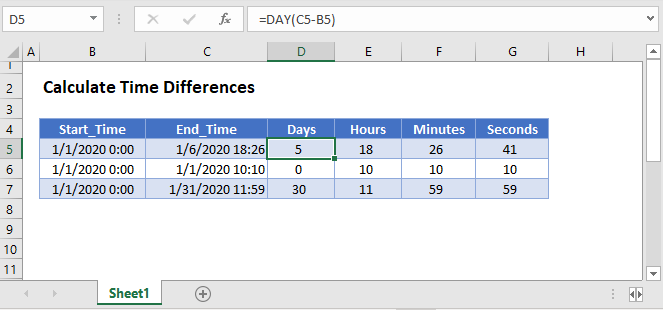## Time Difference

To calculate time differences in Excel simply subtract two times: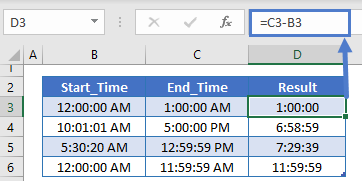Note if you time difference returns a negative number you’ll see this: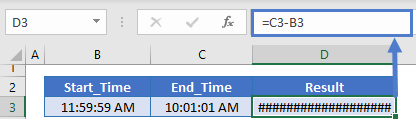To fix the output you can use the ABS Function to calculate the absolute value:

``=ABS(C3-B3)``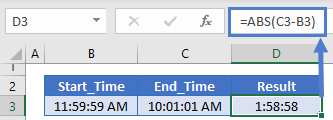### Hours, Minutes, and Seconds Between Times

To display only the hours, minutes, and seconds between times you can use the HOUR, MINUTE, and SECOND Functions: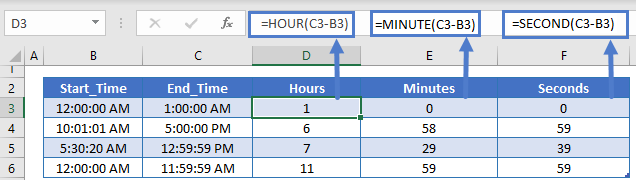### Hours Between Times

To calculate the number of hours between times, subtract the times and multiply by 24 (24 hours in a day). To calculate only the number of full hours use the INT Function:

``=INT((C3-B3)*24)``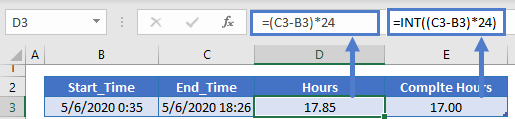### Minutes Between Times

To calculate the number of minutes between times do the same except multiply by 1440 (24 hours * 60 minutes):

``=(C3-B3)*1440``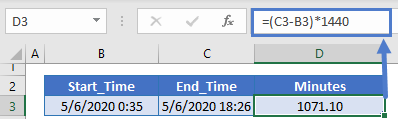### Seconds Between Times

Multiply by 86,400 to calculate the number of seconds between times:

``=(C3-B3)*86400``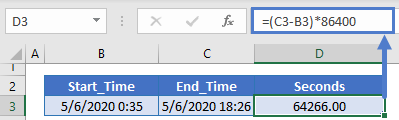### Display Time Difference

You can output a time difference as a string of text using the TEXT Function:

``=TEXT(C3-B3,"hh")``

### Easy Excel Automation## Calculate Time Differences in Google Sheets

All of the above examples work exactly the same in Google Sheets as Excel.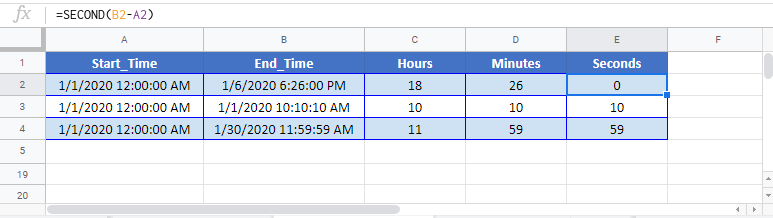### Excel Practice Worksheet

Practice Excel functions and formulas with our 100% free practice worksheets!